Next: SLA_AMP - Apparent to Mean
Up: SUBPROGRAM SPECIFICATIONS
Previous: SLA_AIRMAS - Air Mass

## SLA_ALTAZ - Velocities etc. for Altazimuth Mount

ACTION:
Positions, velocities and accelerations for an altazimuth telescope mount tracking a star (double precision).

CALL:
CALL sla_ALTAZ ( HA, DEC, PHI, AZ, AZD, AZDD, EL, ELD, ELDD, PA, PAD, PADD)

GIVEN:

 D HA hour angle DEC D declination PHI D observatory latitude

RETURNED:

 D AZ azimuth AZD D azimuth velocity AZDD D azimuth acceleration EL D elevation ELD D elevation velocity ELDD D elevation acceleration PA D parallactic angle PAD D parallactic angle velocity PADD D parallactic angle acceleration

NOTES:
1.
Natural units are used throughout. HA, DEC, PHI, AZ, EL and ZD are in radians. The velocities and accelerations assume constant declination and constant rate of change of hour angle (as for tracking a star); the units of AZD, ELD and PAD are radians per radian of HA, while the units of AZDD, ELDD and PADD are radians per radian of HA squared. To convert into practical degree- and second-based units:

 angles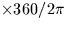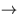degrees velocities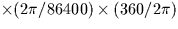degree/sec accelerations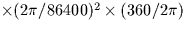degree/sec/sec

Note that the seconds here are sidereal rather than SI. One sidereal second is about 0.99727 SI seconds.

The velocity and acceleration factors assume the sidereal tracking case. Their respective numerical values are (exactly) 1/240 and (approximately) 1/3300236.9.

2.
Azimuth is returned in the range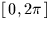; north is zero, and east is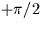. Elevation and parallactic angle are returned in the range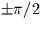. Position angle is +ve for a star west of the meridian and is the angle NP-star-zenith.
3.
The latitude is geodetic as opposed to geocentric. The hour angle and declination are topocentric. Refraction and deficiencies in the telescope mounting are ignored. The purpose of the routine is to give the general form of the quantities. The details of a real telescope could profoundly change the results, especially close to the zenith.
4.
No range checking of arguments is carried out.
5.
In applications which involve many such calculations, rather than calling the present routine it will be more efficient to use inline code, having previously computed fixed terms such as sine and cosine of latitude, and (for tracking a star) sine and cosine of declination.Next: SLA_AMP - Apparent to Mean
Up: SUBPROGRAM SPECIFICATIONS
Previous: SLA_AIRMAS - Air Mass

SLALIB --- Positional Astronomy Library